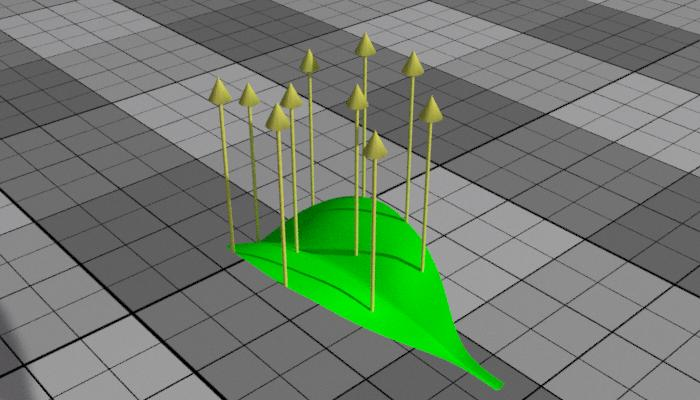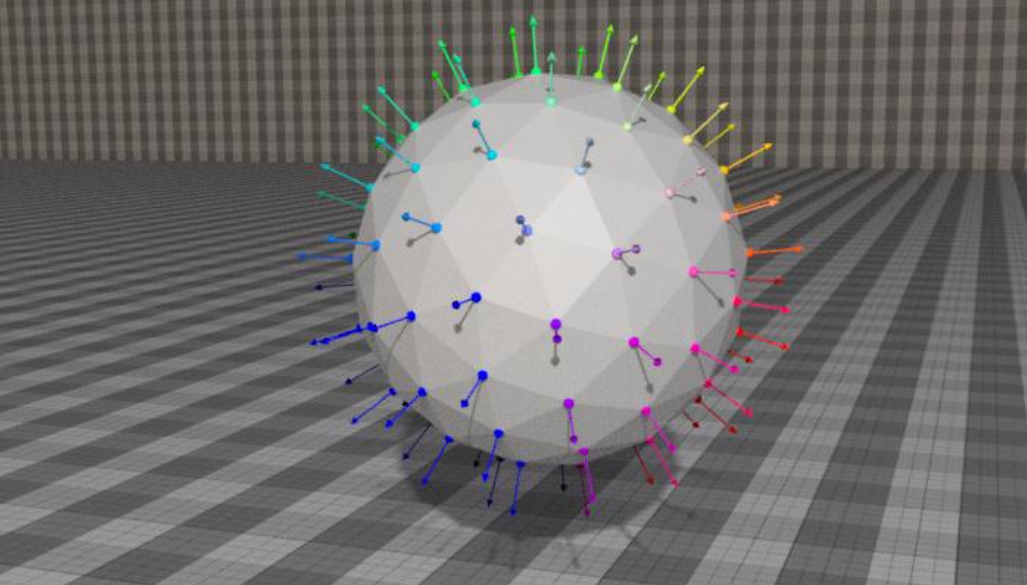# Instance Level Transforms

There are a few attributes, on both RdlInstanceGeometry and TransformSpaceMap , that allow you to transform to and from instance spaces. This guide goes over why and how you would do this.

## What is a Space?

A space is a frame of reference some point or vector is defined in. It is a coordinate system composed of x, y, and z basis vectors, and coordinates are defined relative to that space.

See the example below, where we’re animating the position of the sphere. The texture coordinates are defined relative to world space. Notice that the object swims through the texture.

In object space, coordinates are relative to some object’s local XYZ axes. Below you will see the same example, but where texture coordinates are defined relative to the geometry node’s node_xform. Notice that the texture moves with the object.

Object space does not work with instances, since instances have their own specific transforms. See below, where we have a number of sphere instances, but where the texture coordinates are defined relative to object space.

## Set Instance Levels on Geometry

On RdlInstanceGeometry , you will notice that we have an attribute called instance_level, which has the following options:

• “instance level 0”
• “instance level 1”
• “instance level 2”
• “instance level 3”
• “instance level 4”

This allows you to mark the level or depth of the instance geometry and the reference this with a TransformSpaceMap.

## Render to Instance Space

In order to transform coordinates to instance level space, we can use a TransformSpaceMap , which allows you to transform a point, vector, or normal to/from the desired spaces. For example, we can take transform position P and connect the output to a texture coordinates attribute.

``````
instancer = InstanceGeometry("instancer") {
...
["instance_level"] = "instance level 0"
}

amP = AttributeMap("amP") {
-- position is in render space
["map_type"] = "position"
}

-- transform the points from the AttributeMap to instance level 0 space
tsmSpheres = TransformSpaceMap("tsmSpheres") {
["input"] = bind(amP),
["input_type"] = "point",
["from_space"] = "render",
["to_space"] = "instance level 0"
}

-- use these transformed points as the texture coordinates
noiseMapColor = NoiseMap("noiseMapColor") {
["space"] = "input texture coordinates",
["input_texture_coordinates"] = bind(tsmSpheres),

["frequency_multiplier"] = 4,
["max_level"] = 5,
["use_smoothstep"] = true,
["smoothstep"] = Vec2(0.2, 0.8)
}
``````

If we look at the example from before, we’ll notice that transforming the space to “instance level 0” prevents the texture from “swimming” like it was in object space.

## Maps with Bindable Input

The following maps have bindable input positions and/or normals:

• NoiseMap_v2 and NoiseWorleyMap_v2
``````  ["space"] = "input texture coordinates",
["input_texture_coordinates"] = bind()
``````
• ProjectTriplanarMap_v2 and ProjectTriplanarNormalMap_v2
``````  ["input_position_source"] = "input_position/input_normal",
["input_position"] = bind(),
["input_normal"] = bind()
``````

## Instance Level 1 and Beyond

When adding a second level of instancing (“instance level 1”), the texture on the spheres still sticks. Why does this happen?

TransformSpaceMap concatenates multiple instance levels by default.

• to_space concatenates all of the levels above the specified level. For example, if to_space is “instance level 0”, it would concatenate levels 0, 1, 2, 3, and 4.
• from_space concatenates all of the levels below the specified level. For example, if from_space is “instance level 4”, it would concatenate levels 4, 3, 2, 1, and 0. o
• concatenate_instance_level_transforms allows you to enable/disable this behavior (on by default)

## Instance to Render Space

Let’s say we have a leaf model with custom normals (0, 1, 0), defined in the object space of the model.When you instance these leaves without a TransformSpaceMap, the custom normals still point up throughout the animation.

In this case, you need to transform these normals from the instance’s local space to render space (from_space “instance level 0” to_space “render”).

### Instance Points

Let’s say that the custom normals were defined on the instance points instead of the instanced model.Primitive attributes on instancer points are transferred to the instanced geometry, which means our custom normals are already in “instance level 0” space. However, primitive attributes are not automatically transformed with an object. See below how customN values remain the same, even though their positions are transformed.

There are two ways to handle this issue:

1 Transform our custom normals from the instance’s local space to render space.

``````-- retrieve our custom normals
attrMapCustomN = AttributeMap("attrMapCustomN") {
["map_type"] = "primitive attribute",
["primitive_attribute_name"] = "customN"
}

rotx = frame * 90 / 60

instanceLeavesSphere = InstanceGeometry("instanceLeavesSphere") {
["node_xform"] = rotate(-rotx, 0, 0, 1) * translate(0.0, 4.0, 0.0),
["method"] = "point file",
["point_file"] = "scatter_leaves_sphere.abc", -- our points to scatter leaves on
["references"] = { leaf2Geom },              -- our leaf model
["instance_level"] = "instance level 0",
["use_reference_xforms"] = false
}

-- transform normals from the instance level space to render space
tsmLeavesCustomN = TransformSpaceMap("tsmLeavesCustomN") {
["input"] = bind(attrMapCustomN),
["input_type"] = "vector",
["from_space"] = "object",
["object"] = instanceLeavesSphere,
["to_space"] = "render"
}
``````

2 Use instance level 1. That way, there is no reference to the specific object’s space. Make sure to turn off concatenation of transforms, as we only want the level 1 transform. (Normals are already authored in instance level 0 space)

``````instanceLeavesSphere = InstanceGeometry("instanceLeavesSphere") {
["node_xform"] = rotate(-rotx, 0, 0, 1) * translate(0.0, 4.0, 0.0),
["method"] = "point file",
["point_file"] = "scatter_leaves_sphere.abc", -- our points to scatter leaves on
["references"] = { leaf2Geom },              -- our leaf model
["instance_level"] = "instance level 0"
}

instanceLeafSpheres = InstanceGeometry("instanceLeafSpheres") {
["node xform"] = translate(0.0, 4.0, 0.0),
["method"] = "point file",
["point_file"] = "scatter_leaves_sphere.abc",
["references"] = { sphereGeom, instanceLeavesSphere },
["instance_level"] = "instance level 1"
}

attrMapCustomN = AttributeMap("attrMapCustomN") {
["map_type"] = "primitive attribute",
["primitive_attribute_name"] = "customN"
}

-- transform normals from the instance level space to render space
tsmLeavesCustomN = TransformSpaceMap("tsmLeavesCustomN") {
["input"] = bind(attrMapCustomN),
["input_type"] = "vector",
["from_space"] = "instance level 1",
["to_space"] = "render",
["concatenate_instance_level_transforms"] = false
}
``````